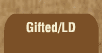Mathematics and Dyslexia

Tips for Learning the Times Tables

Tools for the Times Tables & Workbook

Home > Math > times tables practice >

## The Fives Times Tables

Numbers that you can divide by five always end in 5 or 0. If you multiply an even number by 5, it ends in 0. If you multiply an odd number by 5, the answer ends in 5.

If you multiply any even number by five, the answer is "half of it plus a zero." So, 2 times 5 is half of five (1) with a zero -- making 10. This is because five is half of ten... so if you take half of something and multiply it by ten, you're multiplying by five. (And "when you multiply by ten, stick a zero at the end.") So you can think "5 x 6 ... half of six is 3... thirty" and you're at your answer and you've gotten automatic at taking half of six while you were at it.

So, 5 x 16 is 80 because half of 16 is 8... with a zero is 80.

Practice this page until you can hear these in your mind (or your sleep :)). Say them, see them, and do them. If you're having to count up on your fingers -- you don't know them automatically yet. Trouble getting from 'counting up' to 'automatic answer?' Try these steps:

• First, if the number is more than five, start on finger six with "30." After all, it isn't going to be different. That thumb is just your "thirty" thumb... they even both begin with "th." When you feel yourself starting to count from the start, jump over to that thirty thumb as quick as you can.
• What works for the thumb can work for all those fingers -- the eighth finger is going to be forty every single time. Try going every two fingers and counting by tens to get faster.
1. 5 x 3=
2. 5 x 5=
3. 1 x 5=
4. 5 x 9=
5. 5 x 7=
6. 10 x 5=
7. 6 x 5=
8. 5 x 4=
9. 8 x 5=
10. 5 x 2=
11. 5 x 10=
12. 0 x 5=
13. 7 x 5=
14. 5 x 8=
15. 9 x 5=